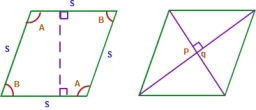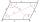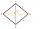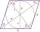# Rhombus and diagonals

The lengths of the diamond diagonals are e = 48cm, f = 20cm. Calculate the length of its sides.

a =  26 cm

### Step-by-step explanation:Did you find an error or inaccuracy? Feel free to write us. Thank you!Tips to related online calculators
Pythagorean theorem is the base for the right triangle calculator.

#### You need to know the following knowledge to solve this word math problem:

We encourage you to watch this tutorial video on this math problem:

## Related math problems and questions:

• The diamondThe diamond has sides of 35 cm and the diagonals are in a ratio of 1: 2. Calculate the lengths of the diagonals.
• Diamond diagonalsCalculate the diamonds' diagonals lengths if the diamond area is 156 cm square, and the side length is 13 cm.
• The diagonalsThe diagonals of the KOSA diamond are 20 cm and 48 cm long. Calculate the circumference of the diamond in centimeters rounded to one unit.
• Two diagonalsThe diagonals of the diamond EFGH have lengths in the ratio 1: 2. What is the circumference of a rhombus if the longer of the diagonals is 8 cm long?
• DiagonalsGiven a rhombus ABCD with a diagonalsl length of 8 cm and 12 cm. Calculate the side length and content of the rhombus.
• Rhombus IVCalculate the length of the diagonals of the rhombus, whose lengths are in the ratio 1: 2 and a rhombus side is 35 cm.
• Two diagonalsThe rhombus has a side length of 12 cm and a length of one diagonal of 21 cm. What is the length of the second diagonal?
• DiagonalsA diagonal of a rhombus is 20 cm long. If it's one side is 26 cm, find the length of the other diagonal.
• RhombusThe rhombus has diagonal lengths of 4.2cm and 3.4cm. Calculate the length of the sides of the rhombus and its height
• Rhombus diagonalsIn the rhombus ABCD are given the sizes of diagonals e = 24 cm; f = 10 cm. Calculate the side length of the diamond and the size of the angles, calculate the content of the diamond
• The diamondThe diamond has an area S = 120 cm2, the ratio of the length of its diagonals is e: f = 5: 12. Find the lengths of the side and the height of this diamond.
• IS trapezoidCalculate the length of diagonal u and height v of isosceles trapezoid ABCD, whose bases have lengths a = |AB| = 37 cm, c = |CD| = 29 cm and legs b = d = |BC| = |AD| = 28 cm.
• Diamond diagonalsFind the diamond diagonal's lengths if the area is 156 cm2 and side is 13 cm long.
• Diamond diagonalsCalculate the diamond's diagonal lengths if its content is 156 cm2 and the side length is 13 cm.
• Diamond and diagonalsA diamond has diagonals f = 8 cm and g = 6 cm long. How long is this diamond perimeter? (Calculate it!)
• A squareA square with a length of diagonals 12cm give: a) Calculate the area of a square b) rhombus with the same area as the square, has one diagonal with a length of 16 cm. Calculate the length of the other diagonal.
• Diamond ABCDIn the diamond ABCD, the diagonal e = 24 cm and the size of angle SAB is 28 degrees, where S is the intersection of the diagonals. Calculate the circumference of the diamond.